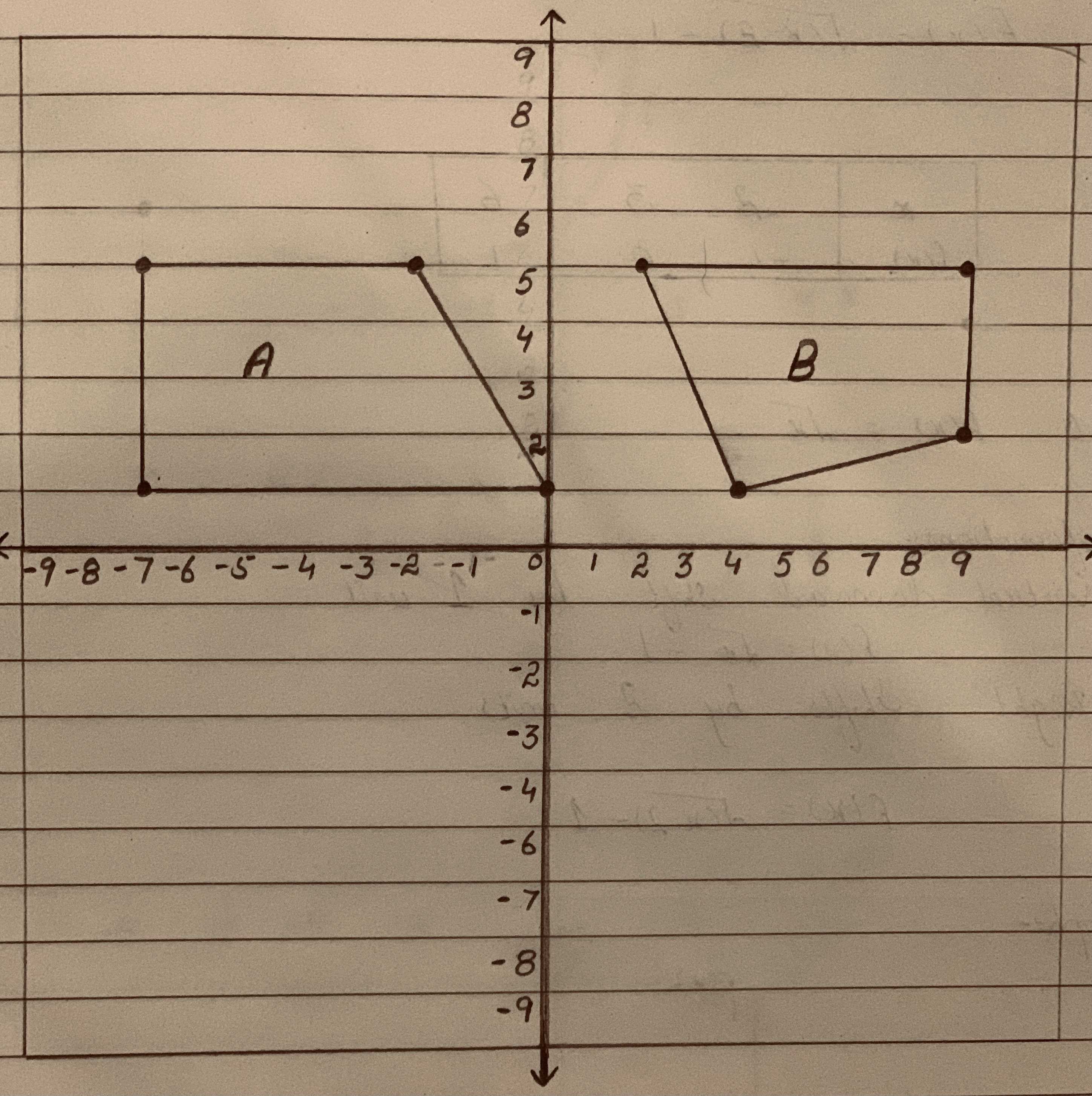banganX

2021-02-03

Lily wants to define a transformation (or series of transformations) using only rotations, reflections or translations that takes Figure A to Figure B.Which statement about the transformation that Lily wants to define is true?
A. It can be defined with two reflections.
B.It can be defined with one rotation and one translation.
C. It cannot be defined because Figure A and Figure B are not congruent.
D.It cannot be defined because the longest side of Figure B is on the bottom.yagombyeR

Step 1

Observe that the quadrilateral in figure A lacks any parallel sides.
The figure B is a quadrilateral with a pair of opposite sides parallel. That is figure B is a trapezium.
The figure A and figure B are not congruent, since A is a quadrilateral and B a trapezium.
Therefore, Figure A cannot be transformed to figure B by using only rotations, reflections or translations.
Step 2

The correct option is C.
Because Figure A and Figure B are identical, it cannot be defined..

Do you have a similar question?